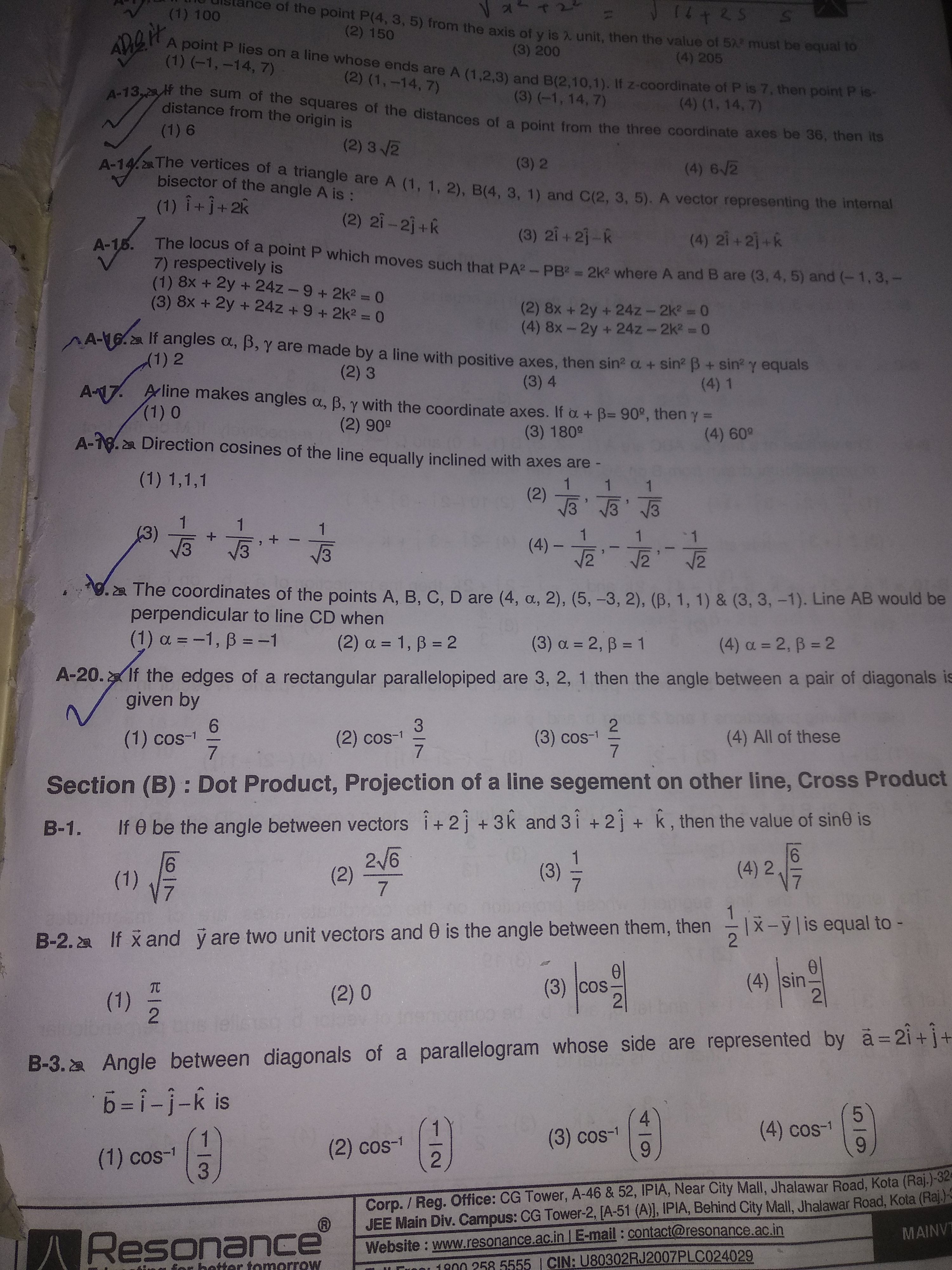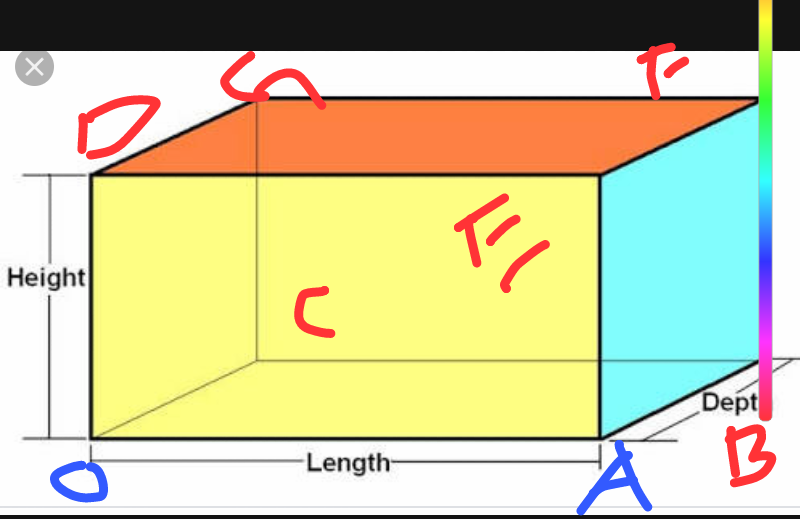# Doubt from 3d geometryA20
Can anyone tell how to visualize there will be 3 different angles between body diagonals?
I can't visulaize this
Also is there any shortcut formulae to these types of question or do we have to find each corner coordinate and than find vector and than find angle?

Wait i am solving with step wise...Point O(0,0,0) F(3,2,1) SO DIAGONAL OF=3i+2j+k
Point A(3,0,0) G(0,2,1) so AG= -3i+2j+k
Now take dot product

Option C

Question not clear ...each face has two diagonal and four longest diagonal...which two diagonal required to find...

brother as tanmay suggested take coordinates of all the cuboidal object assuming left back corner pt as (0,0,0) then find direction ratios of all body diagnols take cos (*inverse) of all these diagnols behold you have your answer
{tip try with fig to solve without writting much}